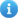COVID-19 | Information and resources to help

# Z39.50 Attributes

Find examples of Z39.50 attributes for targets in Discovery.

The following are same examples of Z39.50 attributes for some targets in Discovery.

See below for information regarding the 'special attributes' used by Index Data.

Innovative (Brown) Use = 1 or u Relation = 2 or r Position = 3 or p Structure =4 or s Truncation = 5 or t Completeness=6 or c
Term attributes 1=1016 2=3 3=3 s=al,4=2 t=r 6=1
Author attributes 1=1003
Title attributes 1=4 2=3 3=3 s=al,s=pw t=l,r 6=1
Subject Attributes 1=21
ISBN attributes 1=7
ISSN attributes 1=8

Innovative (Michigan State) Use = 1 or u Relation = 2 or r Position = 3 or p Structure =4 or s Truncation = 5 or t Completeness=6 or c
Term attributes 1=1016 r=3 p=3 s=pw t=100 c=1
Author attributes 1=1003 r=3 p=3 s=pw t=1 c=1
Title attributes 1=4 r=3 p=3 s=pw t=100 c=1
Subject Attributes 1=21
ISBN attributes 1=7
ISSN attributes 1=8

Aleph (Harvard) Use = 1 or u Relation = 2 or r Position = 3 or p Structure =4 or s Truncation = 5 or t Completeness=6 or c
Term attributes 1=1016
Author attributes 1=1003
Title attributes 1=4
Subject Attributes
ISBN attributes 1=7
ISSN attributes 1=8

Aleph (Umichigan) Use = 1 or u Relation = 2 or r Position = 3 or p Structure =4 or s Truncation = 5 or t Completeness=6 or c
Term attributes 1=1016
Author attributes 1=1003     s=pw
Title attributes 1=4     s=pw
Subject Attributes 1=21
ISBN attributes 1=7
ISSN attributes 1=8

TLC (LYC) Use = 1 or u Relation = 2 or r Position = 3 or p Structure =4 or s Truncation = 5 or t Completeness=6 or c
Term attributes 1=1016
Author attributes 1=1003
Title attributes 1=4
Subject Attributes 1=21
ISBN attributes 1=7
ISSN attributes 1=8

Voyager (Penn) Use = 1 or u Relation = 2 or r Position = 3 or p Structure =4 or s Truncation = 5 or t Completeness=6 or c
Term attributes 1=1016     s=al t=l,r
Author attributes 1=1004     s=al
Title attributes 1=4     s=al
Subject Attributes 1=21     s=al
ISBN attributes 1=7
ISSN attributes 1=8

Voyager (Princeton) Use = 1 or u Relation = 2 or r Position = 3 or p Structure =4 or s Truncation = 5 or t Completeness=6 or c
Term attributes 1=1016     s=al t=l,r
Author attributes 1=1004     s=al
Title attributes 1=4     s=al
Subject Attributes 1=21     s=al
ISBN attributes 1=7
ISSN attributes 1=8

Unicorn (PENNstate) Use = 1 or u Relation = 2 or r Position = 3 or p Structure =4 or s Truncation = 5 or t Completeness=6 or c
Term attributes 1=1016
Author attributes 1=1003
Title attributes 1=4
Subject Attributes 1=21
ISBN attributes 1=7
ISSN attributes 1=8

Horizon (philu) Use = 1 or u Relation = 2 or r Position = 3 or p Structure =4 or s Truncation = 5 or t Completeness=6 or c
Term attributes 1=4
Author attributes 1=1003
Title attributes 1=4
Subject Attributes 1=21
ISBN attributes 1=7
ISSN attributes 1=8

Horizon (Uchicago) Use = 1 or u Relation = 2 or r Position = 3 or p Structure =4 or s Truncation = 5 or t Completeness=6 or c
Term attributes 1=1017
Author attributes 1=1003 r=3   s=1
Title attributes 1=4
Subject Attributes 1=21 r=3   s=2 t=1000
ISBN attributes 1=7
ISSN attributes 1=8

Unicorn (Rutgers) Use = 1 or u Relation = 2 or r Position = 3 or p Structure =4 or s Truncation = 5 or t Completeness=6 or c
Term attributes 1=1016
Author attributes 1=1003
Title attributes 1=4
Subject Attributes 1=21
ISBN attributes 1=7
ISSN attributes 1=8

## Special Attributes used by Index Data

Name Description
s=pw The structure is set to either word or phrase depending on the number of tokens in a term (phrase-word).
s=al Each token in the term is ANDed. (and-list). This does not set the structure at all.
s=ol Each token in the term is ORed. (or-list).  This does not set the structure at all.
r=o Allows ranges and the operators greater-than, less-than, ... equals. This sets Bib-1 relation attribute accordingly (relation ordered). A query construct is only treated as a range if dash is used and that is surrounded by white-space. So -1980 is treated as term "-1980" not <= 1980. If - 1980 is used, however, that is treated as a range.
r=r Similar to r=o but assumes that terms are non-negative (not prefixed with -). Thus, a dash will always be treated as a range. The construct 1980-1990 is treated as a range with r=r but as a single term "1980-1990" with r=o. The special attribute r=r is available in YAZ 2.0.24 or later.
t=l Allows term to be left-truncated. If term is of the form ?x, the resulting Type-1 term is x and truncation is left.
t=r Allows term to be right-truncated. If term is of the form x?, the resulting Type-1 term is x and truncation is right.
t=n If term is does not include ?, the truncation attribute is set to none (100).
t=b Allows term to be both left&right truncated. If term is of the form ?x?, the resulting term is x and truncation is set to both left&right.
t=x Allows masking anywhere in a term, thus fully supporting # (mask one character) and ? (zero or more of any). If masking is used, truncation is set to 102 (regexp-1 in term) and the term is converted accordingly to a regular expression.
t=z Allows masking anywhere in a term, thus fully supporting # (mask one character) and ? (zero or more of any). If masking is used, truncation is set to 104 (Z39.58 in term) and the term is converted accordingly to Z39.58 masking term - actually the same truncation as CCL itself.